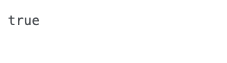# [Java] Assign evaluation result to Java variable

The code to assign the evaluation result to the variable was difficult to understand, so I summarized it to keep my head in order.

## Substitute the evaluation result into a variable

#### `python`

``````
int number = 1;
boolean flag = number == 1;

System.out.println(flag);
``````

The processing result displayed at this time isbecome.
The display result with “0” in number is “false”, but it was difficult to understand, so I supplemented it for myself.

### Decompose boolean flag = number == 1;

#### `python`

``````
/*If number is "1", flag is "ture"*/
if(number == 1){
boolean flag = true;
}
/*If number is "0", flag is "false"*/
else
{
boolean flag = false;
}

``````

Tags:

Updated: